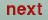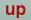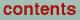Next: Token NGEN= of cast Up: Description of the CTRL Previous: Token X= of cast

## Category SYMGRP (optional)

Provides information to confine integrations to a smaller part of the Brillouin zone. One enters a small number of space group operations; subroutines in the subdirectory SYM will generate all inequivalent products of these operations. For example, the three operations R4X, MX and R3D are sufficient to generate all 48 elements of the cubic octahedron symmetry. Symbols have the form O:(nx,ny,nz) where O is one of M, I or Rj for mirror, inversion and j-fold rotation; and nx,ny,nz are a triplet of indices specifying the axis of operation. You may use X, Y, Z or D as shorthand for (1,0,0), (0,1,0), (0,0,1), (1,1,1). You may also enter products, such as I*R4X. Since these operations restrict numerical integration to a portion of the BZ, it is important that the class definitions in category CLASS are compatible with these operations. If these operations must assume two atoms to be equivalent but are not so defined in CLASS, the electron density in those two atoms may not be equivalent. For non-symmorphic space groups the translational part of the group operation must also be specified (this is only used for calculating the density matrix, i.e. for charge density calculation). This is done by adding after the rotational part :(rx,ry,rz). I. e. for the NiSe2 example above MZ:(0.5,0,0.5). If the token CARTESIAN=F in category OPTIONS the translation is given in terme of the chemists a, b, and c and the rotation part is separated from the translation part by ::. I. e. MZ::(0.5,0,0.5).

O. Jepsen
Thu Oct 12 14:48:45 MESZ 2000